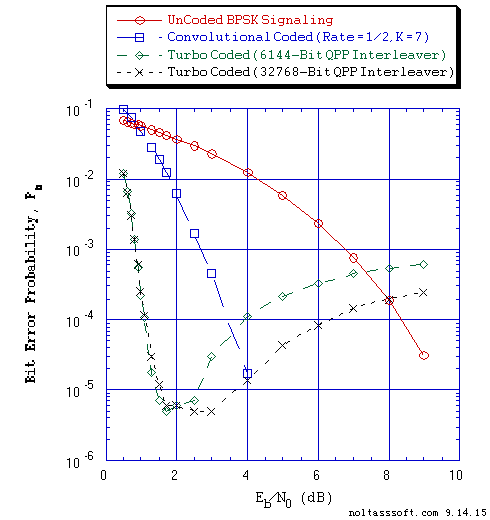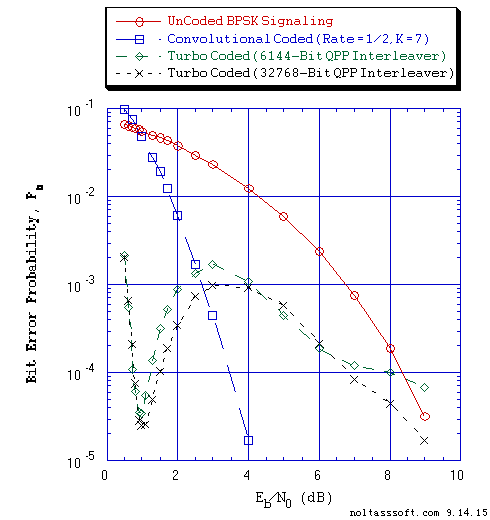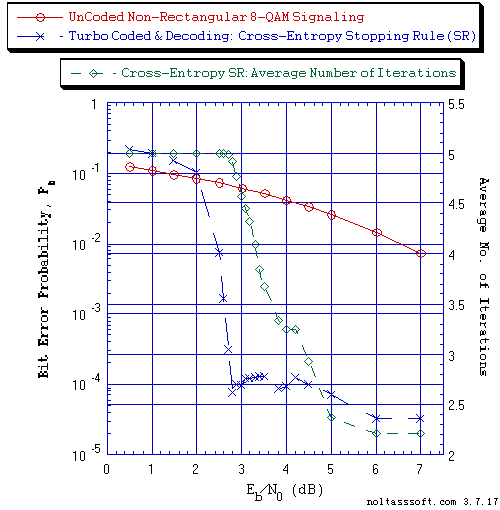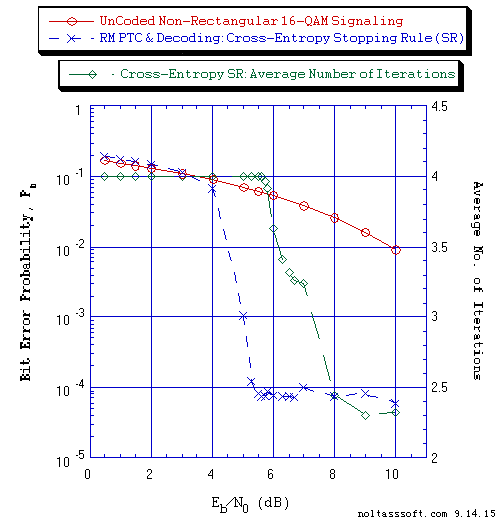# Advanced Turbo Decoding and Iterative Stopping Rule

NOTE: This section is Under-Edit if necessary: Construction began on September 20, 2015 and was finished on September 22, 2015. Update October 10, 2015

Turbo Coded Signaling over a Coherent Memoryless Channel: Iterative Turbo Code Decoder and Cross-Entropy Stopping Rule

```by Darrell A. Nolta
September 22, 2015 with October 10, 2015 Update
```

The AdvDCSMT1DCSS (T1) Professional (T1 Version 2) system tool now offers the capability to model and simulate an Iterative Turbo Code (ITC) Decoder using the Cross-Entropy Stopping Rule (SR). An ITC Decoder Stopping Rule is used to terminate the decoding iteration of each transmitted data block whose size is determined by the Turbo Encoder Interleaver. This feature is in addition to the Fixed Number Stopping Rule used in the Iterative Turbo Decoder to support T1 V2's capability to model and simulate Turbo Coded (TC), Punctured Turbo Coded (PTC) Signaling, and Rate Matching (RM) PTC Signaling over Memoryless Channel (MLC) with Additive White Gaussian Noise (AWGN) or Binary Symmetric MLC (BSC).

As a reminder, the ITC Decoder is a suboptimal iterative decoder that is a serial concatenation of two Soft Input and Soft Decision-Output (SISO) 'symbol-by-symbol' Maximum a Posteriori Probability (MAP) Algorithm component decoders (DEC1 & DEC2). A feedforward path connects DEC1 to DEC2 via an Interleaver. A Feedback Loop connects DEC2 to DEC1 via a DeInterleaver. A Log-MAP or Max-Log-MAP decoding algorithm was used by both component decoders for each simulation case. The ITC Decoder is suboptimal because partial redundant information is feedback to DEC1 from DEC2 (feedforward to DEC2 from DEC1).

The ITC Decoder Fixed Number SR Bit Error Rate (BER) versus signal-to-noise ratio (SNR) Eb/N0 plotted T1 V2 simulated results reveal the behavior of BER instability (i.e., as the Eb/N0 increases and exceeds the BER minimum's Eb/N0, the BER increases) for a given iteration's curve (greater than two iterations). Also, if we compare different iteration curves, we can see BER deterioration. This behavior occurs when the number of iterations exceed the number of iterations required for convergence of the decoding process (i.e., achieve a minimal number of Information Bit Errors). These results lead to the concept of 'over-iterate' where a decoding failure occurs if the ITC Decoder uses too many iterations. This convergence decoding failure is dependent on the value of the signaling process's SNR.

Note that these results correspond to the use of a 6144-bit Quadratic Permutation Polynomial (QPP) Interleaver in the Turbo Encoder and ITC Decoder. Also, the BER instability and deterioration exists in the simulation cases that used the Log-MAP or Max-Log-MAP decoding algorithm in DEC1 and DEC2.

The Turbo Encoding and Turbo Decoding algorithms development in T1 V2 and the concept of Turbo Decoding Convergence was aided by the study of their description in a number of important works. Consult the References section for some of these key works.

This instability effect is an observed simulation result and not an analytical derived determination. Claude Berrou et al. in his 1993 and 1996 papers (, page 1069 & , page 1270) discloses the existence of this instability. Patrick Robertson in his 1994 paper (, page 1302) acknowledges the stability issue but counters the need for Berrou's correction factors. And Shu Lin and Daniel J. Costello, Jr. in their 2004 book (, page 839) discuss the similarity between Iterative Decoding and Negative Feedback in a control system and the possibility of divergence after converging to the correct decision or 'oscillate' between the correct and incorrect decision. This instability effect is real and may be related to the extrinsic information exchange that involves the feedback from DEC2 to DEC1. This instability revelation illustrates the valuable utility of T1 Professional.

First, to investigate the cause of this BER instability, simulated BER results were obtained for Turbo Encoder 32768-bit QPP Interleaver, BPSK Modulation, and ITC Decoding Fixed Number SR using T1 V2.

Consider Figure 1 and 2 for comparison of Turbo Decoding Algorithm BER or Bit Error Probability Pb curves for 6144-bit and 32768-bit QPP Interleavers for 4 Iterations and 6 Iterations, respectively. Observe that the majority of 32768-bit QPP Interleaver cases' BER is smaller than the BER of the 6144-bit QPP Interleaver cases (for the Eb/N0 region where Eb/N0 exceeds the minimum BER's Eb/N0). This result is to be expected given that the larger interleaver action is to increase the randomness (decorrelation) of the two data frame (block) of information bits. But the BER curves still exhibit the BER instability. What is interesting is that for the 6 Iterations cases, we observe that the BER achieves a maximum and then decreases as the Eb/N0 increases in the 'high' Eb/N0 region.

Note additional BER plots for use of the Turbo Encoder 32768-Bit Interleaver and ITC Decoder Fixed Number Stopping Rule (Figure 5 and 6) are shown in the Appendix.

ADVANCED TURBO DECODING and ITERATIVE STOPPING RULE APPENDIX

Next, so to study this behavior of BER instability and 'over-iterate', simulated BER results were obtained using T1 V2 for the Turbo Encoder and ITC Decoder 6144-bit QPP Interleaver and the ITC Decoder utilizing the CE Stopping Rule.

Consider the Turbo Decoding Algorithm BER or Pb performance simulation results that were produced by that are displayed below in Figure 3 plot for Non-Rectangular 8-QAM Modulation Scheme (Turbo Coded Signaling) and Figure 4 plot for Non-Rectangular (NR) 16-QAM Modulation Scheme (Rate Matching Punctured Turbo Coded Signaling). Also, the Average Number of Iterations corresponding to each data point (Eb/N0, Pb) is shown in each figure.

The 8-QAM Non-Rectangular signal vector locations (constellation) match the non-square 8-QAM constellation locations in a 2002 IEEE 802.16 Broadband Wireless Access Work Group proposal . The NR 16-QAM constellation is derived from the Square 16-QAM to form a cross shaped constellation. Consult Figure 1 of reference  for depiction of this NR 16-QAM constellation.

We observe that there is a dramatic decrease in the BER instability as the Eb/N0 is increased beyond the BER minimum's Eb/N0 in Figure 3 and 4 BER plots. Also, the Average Number of Iterations decrease from the maximum number of iterations of 5 and 4 for Figure 3 and Figure 4, respectively. But we observe in Figure 3 (Figure 4) a small (smaller) BER oscillation in the region of dramatic decrease of the Average Number of Iterations before the BER begins to decrease again.

Note additional BER plots for Turbo Coded Signaling using the 6144-Bit Interleaver in the Turbo Encoder and the Cross-Entropy Stopping Rule in ITC Decoder (Figure 7, 8, 9, 10, 11, 12, 13, and 14) are shown in the Appendix: Figure 7 (Figure 12) and Figure 8 (Figure 13) plots for BPSK Modulation (TC and PTC Signaling), Figure 9 plot for Non-Rectangular 8-QAM Modulation Scheme (TC Signaling), Figure 10 (Figure 14) plot for QPSK Modulation Scheme (PTC Signaling), and Figure 11 for Non-Rectangular 16-QAM Modulation Scheme (RM PTC Signaling).

ADVANCED TURBO DECODING and ITERATIVE STOPPING RULE APPENDIX

We have derived from these T1 V2 Turbo Coded Signaling and Iterative Turbo Code Decoding simulated BER results the following observations:

The BER instability and deterioration is real and is dependent on the Turbo Encoder and ITC Decoder Interleaver block (frame) size, channel signaling SNR (Eb/N0), and the ITC Decoding's number of iterations.

Each BER plot illustrates the performance advantage of using the CE Stopping Rule in the Iterative Turbo Code Decoder for the purpose of halting the decoding process for each transmitted data block. The CE Stopping Rule for each data block decoding prevents the 'over-iteration' of that data block. Note that "The CE stopping rule is based on the difference between the a posteriori L-values after successive iterations at the outputs of the two decoders" according to Lin and Costello, Jr. (, page 841). The halting process occurs when the CE function drops below its threshold.

The simulated CE Stopping Rule results imply that the extrinsic information used by DEC1 and DEC2 for a given iteration (beyond the convergent iteration) causes the loss of a data block convergence, i.e., the ITC Decoder starts to make incorrect decisions (estimates) on a transmitted block's data bits (information bits) after making correct decisions on these data bits (information bits).

One must remember that key to the successful operation of an ITC Decoder is that the inputs [channel output signal (s) and extrinsic information signal] to DEC1 (DEC2) are statistically independent (uncorrelated) to each other. This means that the corruption of each signal must not become correlated during the iterative process. For 'high' SNR signaling over an AWGN MLC, a channel output signal will have little uncorrelated noise relative to the channel input signal.

Let us focus on the nature of the ITC Decoder, i.e. its decoding algorithm is suboptimal globally even though the individual decoders are operating optimally. This fact means that there is not a unique solution to the Turbo Decoding problem, i.e., the composite decoder chooses a block of Estimated Data (Information) bits out of a set of possible Estimated Data Blocks. The CE Stopping Rule halts the decoding for a block when Convergence has occurred for the block of bits and not for each bit. Convergence does not imply optimality of an estimated data block choice.

In conclusion, the real nature of Turbo Decoding is actually an Optimization problem, not a Communications System problem. Given the importance of Iterative Decoding, the problem of why the feedback (& associated feedforward) of extrinsic likelihood (information) signal causes the BER instability and deterioration needs to be address from an Optimization perspective in addition to the use of simulation. The implementation of the Cross-Entropy Stopping Rule in the ITC provides a practical solution to this problem but adds complexity and associated cost to Turbo Decoding.

T1 Professional (T1 V2) is an invaluable system tool in its use to study important behavior found in the use of Suboptimal Iterative Decoding in Digital Communications Systems.```Figure 1. Bit Error Probability for UnCoded, Rate = 1/2 Convolutional Coded, and
Rate = 1/3 Turbo Coded (using a QPP Interleaver & 4-Iteration Turbo
Decoder) BPSK Signaling over a Coherent Memoryless Channel with Additive
White Gaussian Noise (AWGN):
```
```          Equal probable Independent and Identical Distributed  (IID) Source for
10 Million, 1 Million, and 1,001,472 Information Bits for UnCoded,
Convolutional Coded, and Turbo Coded BPSK Signaling respectively
over a Vector Channel;
```
```          Rate = 1/2, Constraint Length K = 7 (3,1,0,3,3,2,3) a Best (Optimal)
Non-Recursive Convolutional Code (J.P. Odenwalder) and Viterbi Algorithm
using a Path Memory Length of 35 bits and an Unquantized Branch Metric;
```
```          Rate (r) = 1/3, K = 3 Turbo Code based on a r = 1/2, K = 3 (G0 = 7
octal, G1 = 5 octal) Recursive Systematic Convolutional component
code and a 6144-Bit or 32768-Bit QPP Turbo Encoder Interleaver; and
```
```          Log-MAP Iterative Turbo Decoder using Systematic Channel Output as
input to both component decoders and Decode Information Bit Decision
is based on the Sum of both decoders' log-likelihood ratios (L-values)
and for Four Iterations Fixed Number Stopping Rule.
``````Figure 2. Bit Error Probability for UnCoded, Rate = 1/2 Convolutional Coded, and
Rate = 1/3 Turbo Coded (using a QPP Interleaver & 6-Iteration Turbo
Decoder) BPSK Signaling over a Coherent Memoryless Channel with Additive
White Gaussian Noise (AWGN):
```
```          Equal probable Independent and Identical Distributed  (IID) Source
for 10 Million, 1 Million, and 1,001,472 Information Bits for UnCoded,
Convolutional Coded, and Turbo Coded BPSK Signaling respectively over
a Vector Channel;
```
```          Rate = 1/2, Constraint Length K = 7 (3,1,0,3,3,2,3) a Best (Optimal)
Non-Recursive Convolutional Code (J.P. Odenwalder) and Viterbi
Algorithm using a Path Memory Length of 35 bits and an Unquantized
Branch Metric;
```
```          Rate (r) = 1/3, K = 3 Turbo Code based on a r = 1/2, K = 3 (G0 = 7
octal, G1 = 5 octal) Recursive Systematic Convolutional component
code and a 6144-Bit or 32768-Bit QPP Turbo Encoder Interleaver; and
```
```          Log-MAP Iterative Turbo Decoder using Systematic Channel Output as input
to both component decoders and Decode Information Bit Decision is based
on the Sum of both decoders' log-likelihood ratios (L-values) and
for Six Iterations Fixed Number Stopping Rule.
``````Figure 3. Bit Error Probability for UnCoded and Rate = 1/3 Turbo Coded (TC)
Non-Rectangular 8-QAM Signaling over a Coherent Memoryless Channel
with Additive White Gaussian  Noise (AWGN):
```
```          Equal probable Independent and Identical Distributed (IID) Source
for 10,000,002 and 1,001,472 Information Bits for UnCoded, and Turbo Coded
Non-Rectangular (NR) 8-QAM Signaling respectively over a Vector Channel;
```
```          Rate (r) = 1/3, K = 4 Turbo Code based on a r = 1/2, K = 4
G0 = 13 octal, G1 = 17 octal) Recursive Systematic Convolutional
component code (Optimum-weight Spectrum) and a 6144-Bit QPP Turbo Encoder
Interleaver;
```
```          NR 8-QAM Demodulation Constellation DeMapper Algorithm: Max-Log Bit
Metrics; and
```
```          Max-Log-MAP Iterative Turbo Decoder using Systematic Channel Output
as input to both component decoders and Decode Information Bit Decision
is based on the Sum of both decoders' log-likelihood ratios
(L-values) and for Cross-Entropy (5 Iterations Maximum)
Stopping Rule.
``````Figure 4. Bit Error Probability for UnCoded and Rate Matching (RM) Punctured
Turbo Coded (PTC) 'Impure' Gray Coded Non-Rectangular (NR) 16-QAM
Signaling over a Coherent Memoryless Channel with Additive White
Gaussian Noise (AWGN):
```
```          Equal probable Independent and Identical Distributed (IID) Source
for 10 Million and 1,001,472 Information Bits for UnCoded and RM PTC
Gray Coded NR 16-QAM Signaling respectively over a Vector Channel;
```
```          Rate (r) = 1/3, K = 4 Parent Turbo Code based on a r = 1/2, K = 4 (G0
= 13 octal, G1 = 17 octal) Recursive Systematic Convolutional component
code (Optimum-weight Spectrum) and a 6144-Bit QPP Turbo Encoder
Interleaver;
```
```          Punctured Turbo Code Rate = 1/2 for Puncturing Period of 2 and
Puncturing Matrix P = [[1,1],[1,0],[0,1]];
```
```          'Impure' Gray Code NR 16-QAM Demodulation Constellation DeMapper
Algorithm: Max-Log Bit Metrics; and
```
```          Max-Log-MAP Iterative Turbo Decoder using Systematic Channel
Output as input to both component decoders and Decoder Information
Bit Decision is based on the Sum of both decoders' log-likelihood
ratios (L-values) and for Cross-Entropy (4 Iterations Maximum) Stopping
Rules.
```
References:

 Claude Berrou, Alain Glavieux, and Punya Thitimajshima, "Near Shannon Limit Error-Correcting Coding: Turbo -Codes (1)," in Proc., 1993 IEEE International Conference on Communications (Geneva, Switzerland), pp. 1064-1070, May 1993.

 Patrick Robertson, "Illuminating the Structure of Code and Decoder of Parallel Concatenated Recursive Systematic (Turbo) Codes," in Proc., IEEE Globecom Conference (San Francisco, CA), pp. 1296-1303, December 1994.

 Patrick Robertson, Emmanuelle Villebrun, and Peter Hoeher, "A Comparison of Optimal and Sub-Optimal MAP Decoding Algorithms Operating in the Log Domain," in Proc. IEEE ICC'95, Seattle, WA, pp. 1009-1013, June 1995.

 Shlomo Shamai (Shitz) and Sergio Verdu, "An Information Theoretic Approach to Turbo Codes," 1996 Mediterranean Workshop on Coding and Information Integrity, Palma de Mallorca, Spain, February 1996.

 Sergio Benedetto and Guido Montorsi, "Unveiling Turbo Codes: Some Results on Parallel Concatenated Coding Schemes," IEEE Transactions on Information Theory, Vol. 42, No. 2, pp. 409-428, March 1996.

 Joachim Hagenauer, Elke Offer, and Lutz Papke, "Iterative Decoding of Binary Block and Convolutional Codes," IEEE Transactions on Information Theory, Vol. 42, No. 2, pp. 429-445, March 1996.

 Patrick Robertson and Thomas Worz, "A Novel Bandwidth Efficient Coding Scheme Employing Turbo Codes," in Proc. of 1996 IEEE International Conference on Communications (Dallas, TX), ICC '96, pp. 962-967, June 1996.

 Claude Berrou and Alain Glavieux, "Near Optimum Error Correcting Coding and Decoding: Turbo Codes," IEEE Transactions on Communications, Vol. 44, No. 10, pp. 1261-1271, October 1996.

 Lance C. Perez, Jan Seghers, and Daniel J. Costello, Jr., "A Distance Spectrum Interpretation of Turbo Codes," IEEE Transactions on Information Theory, Vol. 42, No. 6, pp. 1698-1709, November 1996.

 Robert J. McEliece, David J.C. MacKay, and Jung-Fu Cheng, "Turbo Decoding as an Instance of Pearl's "Belief Propagation" Algorithm," IEEE Journal on Selected Areas in Communications, Vol. 16, No. 2, pp. 140-152, February 1998.

 S.A. Barbulescu, "Dynamical system perspective on turbo codes," Electronics Letters, Vol. 34, No. 8, pp. 754-755, April 16, 1998.

 M.C. Valenti and J. Sun, "The UMTS Turbo Code and an Efficient Decoder Implementation Suitable for Software-Defined Radios," International Journal of Wireless Information Networks, Vol. 8, No. 4, October 2001.

 Stephan ten Brink, "Convergence Behavior of Iteratively Decoded Parallel Concatenated Codes," IEEE Transactions on Communications, Vol. 49, No. 10, pp. 1727-1737, October 2001.

 J.A. Torres, "Method for using non-squared QAM constellations", IEEE 802.16 Broadband Wireless Access Working Group, http://www.ieee802.org/16/tga/contrib/C80216a-02_66.pdf, May 2002.

 Shu Lin and Daniel J. Costello, Jr., Error Control Coding: fundamentals and applications, Second Edition, Pearson Prentice Hall, Upper Saddle River, New Jersey, 2004.

 D.A. Nolta, "Turbo Coded Signaling over a Coherent Memoryless Channel: Rate Matching and the Iterative Turbo Decoder," http://www.noltasssoft.com, May 2014.

 Advanced Turbo Decoding and Iterative Stopping Rule Appendix